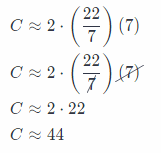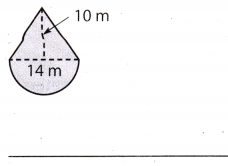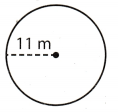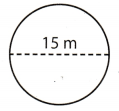Refer to our Texas Go Math Grade 7 Answer Key Pdf to score good marks in the exams. Test yourself by practicing the problems from Texas Go Math Grade 7 Module 9 Quiz Answer Key.

9.1 Angle Relationships

Use the diagram to name a pair of each type of angle.

Question 1.
Supplementary angles _____
∠DFC and ∠BFC

Go Math Module 9 Answer Key Module 9 Test Question 2.
Complementary angles. _____
∠AFB and ∠BFC

Question 3.
Vertical angles. _____
∠EFD and ∠BFC

9.2, 9.3 Finding Circumference and Area of Circles

Find the circumference and area of each circle. Use 3.14 for π.Question 4.
Circumference ≈ _____
d = 36 cm
Use the formula for the circumference of the circle when given diameter
C = π(d) Substitute 36 for d, and 3.14 for π.
C ≈ 3.14(36)
C ≈ 113.04
The circumference of the circle is 113.04 cm
C ≈ 113.04 cm

Question 5.
area ≈ ___
Use the formula for the area of the circle.
Since the diameter is twice a radius, the formula for area of a circle, when given the diameter, is
A = π$$\left(\frac{d}{2}\right)^{2}$$ Substitute 36 for d, and 3.14 for π.
A ≈ 3.14 . $$\left(\frac{36}{4}\right)^{2}$$
A ≈ 3.14 182
A ≈ 3.14 . 324
A ≈ 1017.36
The area of the circle is about 1017.36 cm2.

A ≈ 1017.36 cm2Question 6.
Circumference ≈ _____
The radius of the circle is 7 m.
Use the formula for the circumference of the circle.
C = 2π(r) Substitute 7 for r, and $$\frac{22}{7}$$ for π.The circumference is about 44 m.

C ≈ 44

area ≈ ___
Use the formula for the area of the circle
A = Substitute 7 for r, and 314 for w.
A ≈ 3.14(7)2
A ≈ 3.14 49
A ≈ 153.86
The area of the circle is about 153.86 m2

A ≈ 153.86 m2.

9.4 Area of Composite Figures

Find the area of each figure. Use 3.14 for π.

Question 8.Separate the figure into a triangle and one semicircle.
Area of the triangle
base = 14 m
height = 10 m
Use the formula for the area of the triangle.
A1 = $$\frac{1}{2} b \cdot h$$
A1 = $$\frac{1}{2} 14 \cdot 10$$
A1 = $$\frac{70}{2}$$
A1 = 35
The area of the triangle is 35 m2.

Area of the circle
diameter = 14 m
Use the formula for the area of the circle when given the diameter
Ac = $$\pi\left(\frac{d}{2}\right)^{2}$$ Subsitute 14 for d and 3.14 for π.
Ac = 3.14$$\left(\frac{14}{2}\right)^{2}$$
Ac = 3.14 . 72
Ac = 3.14 . 49
Ac = 153.86
Area of the semicircle is half the area of the circle.
A2 = $$\frac{A_{c}}{2}$$ = $$\frac{153.86}{2}$$ = 76.93
The area of the semicircle is 76.93 m2.
Add the areas to find the total area.
A = A1 + A2 = 35 + 76.93 = 111.93
The area of the figure is 111.93 m2.

Quiz for Grade 7 Math Module 9 Question 9.Separate the figure into a rectangle and a parallelogram.
Area of the rectangle
length = 20 cm
width = 5.5 cm
Use the formula for the area of the rectangle.
A1 = l . w
A1 = 20 . 5.5
A1 = 110
The area of the rectangle is 110 cm2.
Area of the parallelogram
b = 20 cm
h = 4.5 cm
Use the formula for the area of the parallelogram.
A2 = b . h
A2 = 20 . 4.5
A2 = 90
The area of the parallelogram is 90 cm2

Add the areas to find the total area.
A = A1 + A2 = 110 + 90 = 200
The area of the figure is 200 cm2.

Essential Question

Question 10.
How can you use geometric formulas in real-world situations?
There are times that we need to determine the area perimeter or volume of a certain object or even a place and
the only given is the dimensions, the geometric formulas are very helpful in such a way that we can calculate it by ourselves.

The geometric formulas are very helpful.

Texas Go Math Grade 7 Module 9 Mixed Review Texas Test Prep Answer Key

Selected Response

Use the diagram for Exercises 1-3.Question 1.
What is the measure of ∠BFC?
(A) 18°
(B) 72°
(C) 108°
(D) 144°
(C) 108°

Explanation:
∠AFB and ∠BFC are supplement angles, hence
∠AFB + ∠BFC = 180° Substitute 72° for ∠AFB
72° + ∠BFC = 180° Subtract 72° from both sides.
∠BFC = 180° – 72°
∠BFC = 108°

Math Quiz for Grade 7 Module 9 Test Question 2.
Which describes the relationship between ∠BFA and ∠CFD?
(B) complementary angles
(C) supplementary angles
(D) vertical angles
(D) vertical angles

Question 3.
Which information would allow you to identify ∠BFA and ∠AFE as complementary angles?
(A) m∠AFE = 108°
(B) ∠DFE is a right angle.
(C) ∠BFA and ∠BFC are supplementary angles.
(D) ∠BFA and ∠BFC are adjacent angles.
(B) ∠DFE is a right angle.

Explanation:
∠DFE is a right angle, as we see in the diagram.

Question 4.
David pays $7 per day to park his car. He uses a debit card each time. By what amount does his bank account change due to parking charges over a 40-day period? (A) -$280
(B) -$47 (C)$47
(D) $280 Answer: (A) -$280

Explanation:
David pays $7 each day for 40 days, so amount on his bank account decreases for$7 . 40 = \$280.

Question 5.
What is the circumference of the circle? Use 3.14 for π.(A) 34.54 m
(B) 69.08 m
(C) 379.94 m
(D) 1,519.76 m
(B) 69.08 m

Explanation:
r = 11 m
Use the formula for circumference.
C = 2πr(r) Substitute 11 for r and 314 for π
C ≈ 2 3.14 . 11
C ≈ 69.08
The circumference of the circle is 69.08 m.

What is the area of the circle? Use 3.14 for π.(A) 23.55 m2
(B) 176.625 m2
(C) 47.1 m2
(D) 706.5 m2
(B) 176.625 m2

Explanation:
d = 15m
Use the formula for the area of the circle.
A = πr2
Since the diameter is twice a radius, the formula for area of a circle, when given the diameter, is
A = π(r)2 Substitute $$\frac{d}{2}$$ for r.
A = $$\pi\left(\frac{d}{2}\right)^{2}$$ Substitute 15 for d, and 3.14 for π.
A ≈ 3.14 . $$\left(\frac{15}{2}\right)^{2}$$
A ≈ 3.14 . (7.5)2
A ≈ 3.14 . 56.25
A ≈ 176.62
The area of the circle is about 176.62 m2.

Gridded Response

Find the area in square meters of the figure below. Use 3.14 for π.Separate the figure into a square and a quarter of circle.
Area of the square
side = 6 m
Use the formula for the area of the square
A1 = 82
A1 = 62
A1 = 36
The area of the square is 36 m2.
To find the area of the quarter of a circle, find the area of the circle and divide it by 4.
Area of the circle
A2 = $$\frac{A_{c}}{4}$$ = $$\frac{113}{404}$$ = 28.26